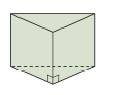Chapter 9.1, Problem 8E### Elementary Geometry for College St...

6th Edition
Daniel C. Alexander + 1 other
ISBN: 9781285195698

#### Solutions

Chapter
Section### Elementary Geometry for College St...

6th Edition
Daniel C. Alexander + 1 other
ISBN: 9781285195698
Textbook Problem
1 views

# Suppose that each of the bases of the triangular prism in Exercise 2 has an area of 3.4 in2 and that each lateral face has an area of 4.6 in2. Find the total (surface) area of the prism.To determine

To Find:

The total (surface) area of the prism.

Explanation

The total area of any solid is the area of all the number of lateral sides that it have, including the area of the top and the bottom of solid.

Thus, the total area (T) of any solid prism with its lateral area (L) and base area (B) is given by the formula.

T=L+2B

Here, we have 2B since, any solid prism will have two bases (One upper part and another the lower part).

Calculation:

Given,

The base area of the triangular prism (each) =3.4 in2.

The lateral face area of the triangular prism (each) =4.6 in2.

Thus, here we have

B=3.4 in2. and

But the lateral area ‘L’ of the triangular prism is given by multiplying the area of a lateral face of the prism by 3 since, a triangular prism has three lateral faces of same area

### Still sussing out bartleby?

Check out a sample textbook solution.

See a sample solution

#### The Solution to Your Study Problems

Bartleby provides explanations to thousands of textbook problems written by our experts, many with advanced degrees!

Get Started